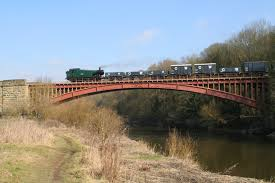# Would You Travel in a Goods Train?A Goods Train crosses a $\SI{120}{\metre}$ long bridge with uniform speed $v$ in $t$ seconds.

Then, if it were to cross a $\SI{240}{ \metre}$ long bridge with uniform speed $2v$, it would require ______

×